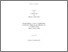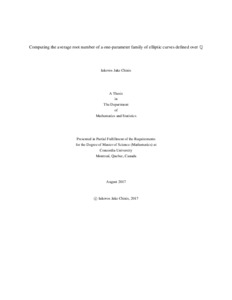Title:

# Computing the average root number of a one-parameter family of elliptic curves defined over Q

Chinis, Iakovos Jake (2017) Computing the average root number of a one-parameter family of elliptic curves defined over Q. Masters thesis, Concordia University.Preview Text (application/pdf) Chinis_MSc_F2017.pdf - Accepted Version Available under License Spectrum Terms of Access. 308kB

## Abstract

The root number $w$ of an elliptic curve defined over $\Q$ has an intrinsic definition as an infinite product of \textit{local root numbers} $w_p$, over all places $p$ of $\Q$, with $w_p=\pm 1$ for all $p$ and such that $w_p=1$ for all but finitely-many $p$. By considering a one-parameter family of elliptic curves defined over $\Q$, we might ask ourselves if there is any bias in the distribution (or parity) of the root numbers at each specialization.

From the work of Helfgott in his Ph.D. thesis, we know (at least conjecturally) that the average root number of an elliptic curve defined over $\Q(T)$ is zero as soon as there is a place of multiplicative reduction over $\Q(T)$ other than $-\deg$.

In this paper, we are concerned with elliptic curves defined over $\Q(T)$ with no place of multiplicative reduction over $\Q(T)$, except possibly at $-\deg$. More precisely, we use the work of Helfgott to compute the average root number of an explicit family of elliptic curves defined over $\Q$ and show that this family is "parity-biased" infinitely-often.

Divisions: Concordia University > Faculty of Arts and Science > Mathematics and Statistics Thesis (Masters) Chinis, Iakovos Jake Concordia University M. Sc. Mathematics August 2017 David, Chantal 982852 Iakovos Chinis 16 Nov 2017 17:34 18 Jan 2018 17:55
All items in Spectrum are protected by copyright, with all rights reserved. The use of items is governed by Spectrum's terms of access.

Repository Staff Only: item control pageResearch related to the current document (at the CORE website)
Back to top